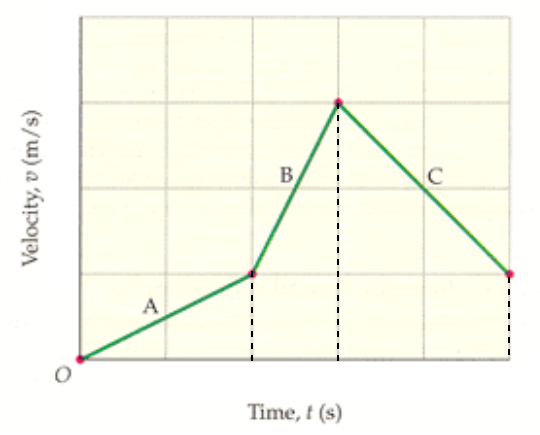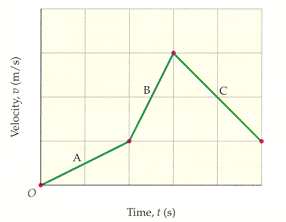# Problem: A person on horseback moves according to the velocity-versus-time graph shown below. (The vertical axis is marked in increments of 2 m/s and the horizontal axis is marked in increments of 5 s.) Find the displacement of the person for each of the segments A, B, and C individually, as well as the whole trip represented by the graph.m (A)m (B)m (C)m (whole trip)

###### FREE Expert Solution

For a velocity-versus-time graph, the displacement is the area under the curve.

For the three regions, we can visualize their area from the figure below.We see that region A is in the shape of a triangle while regions B and C are in the shapes of trapezium.

The area of a triangle is given by:

$\overline{){\mathbf{A}}{\mathbf{=}}\frac{\mathbf{1}}{\mathbf{2}}{\mathbf{b}}{\mathbf{h}}}$, where b is the base and h is the perpendicular height.

79% (311 ratings)###### Problem Details

A person on horseback moves according to the velocity-versus-time graph shown below. (The vertical axis is marked in increments of 2 m/s and the horizontal axis is marked in increments of 5 s.) Find the displacement of the person for each of the segments A, B, and C individually, as well as the whole trip represented by the graph.m (A)
m (B)
m (C)
m (whole trip)

Frequently Asked Questions

What scientific concept do you need to know in order to solve this problem?

Our tutors have indicated that to solve this problem you will need to apply the Calculating Displacement from Velocity-Time Graphs concept. You can view video lessons to learn Calculating Displacement from Velocity-Time Graphs. Or if you need more Calculating Displacement from Velocity-Time Graphs practice, you can also practice Calculating Displacement from Velocity-Time Graphs practice problems.